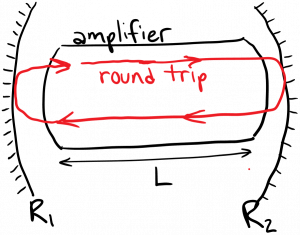Laser Photonics Lasing and Threshold

# Lasing and Threshold

We’ve finally assembled all of the tools we need to being discussing lasers.  We said that an optical amplifier is an inverted material or ‘gain medium’ which amplifies light using stimulated emission.  As discussed at the very beginning, to construct a laser, we add optical feedback to an optical amplifier.  The easiest way (but not the only way) to accomplish this is to place a mirror at either end of the amplifier, thus enclosing the amplifier in a Fabry-Perot cavity.

In order to ‘turn on’ the laser, we must begin pumping the amplifier (via discharge, optically, or other pumping mechanism). When do we consider the laser to be ‘on’?   If we pump the amplifier too weakly, the population of atoms in the amplifier won’t be inverted, resulting in very little stimulated emission.  In this case the laser isn’t properly ‘on’.  All lasers have a minimum pump rate that is necessary to establish lasing: the condition where the output light is dominated by stimulated emission ‘copies’ of the same photon.  The minimum pump rate necessary to achieve lasing is called the threshold condition.

Reaching threshold involves satisfying two requirements.  The first requirement is achieving inversion in the gain medium (amplifier).  But inversion alone isn’t sufficient.  The second requirement is to establish a light beam within the cavity which is sustained indefinitely by stimulated emission.  Let’s imagine a photon that is spontaneously emitted within the laser.  As this new photon propagates, it is amplified (copied) by stimulated emission.   The resulting light beam then strikes a mirror, where some of its photons are lost to transmission (or scattering, or absorption).  If we consider a round-trip for the beam within the laser cavity, the beam undergoes both amplification (gain) and loss.  If the total loss on a round-trip is greater than the total gain, the light beam will lose intensity after each round-trip it takes; it won’t be sustained indefinitely.   Other spontaneously emitted photons will meet the same fate.  However, if the round trip gain exactly balances the loss, the beam will be sustained indefinitely by stimulated emission: we have achieved threshold!  The above-threshold light output from the laser will have a narrower spectrum and higher coherence and intensity compared to below-threshold light output, because it is almost entirely due to stimulated emission ‘copies’ of a single photon.Let’s try to write this threshold condition mathematically.  What happens to the intensity of the beam on a round-trip in the cavity diagrammed above?  It reflects twice and is amplified over a distance equal to twice the amplifier length.  Therefore, the intensity of the beam after a round-trip is related to the intensity of the beam before a round-trip by:$\displaystyle I_{after} = R_1^2 e^{\gamma L} R_2^2 e^{\gamma L} I_{before}$

where$R_1$ and$R_2$ are the reflection coefficients of mirror 1 and 2, respectively (squaring them gives the power reflectance).  A beam at threshold must be sustained by stimulated emission on one or more round-trips, meaning$I_{after} = I_{before}$.  Therefore the threshold gain$\gamma_{th}$ must satisfy$\displaystyle R_1^2 R_2^2 e^{2 \gamma_{th} L} = 1$

or$\displaystyle \gamma_{th} = -\frac{1}{L} \ln {(R_1 R_2)}$

Considering this for a moment, it tells us that the threshold gain per length is reduced if we increase$L$ or if we increase$R_1, R_2$.  (Why?)  If additional losses are present in the laser cavity, they will have to be added to the right hand side of this equation.

The pump rate necessary to achieve the threshold gain is the threshold pump rate$R_{th}$.  We’ll calculate this pump rate for certain cases later on.